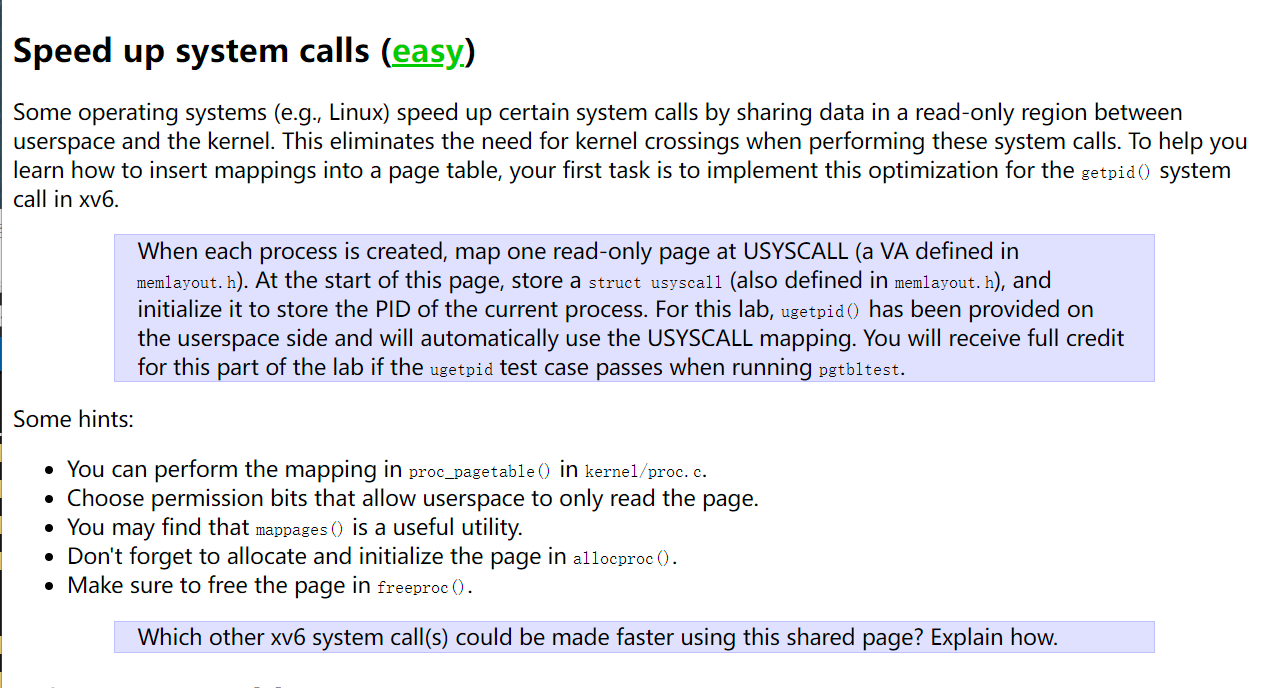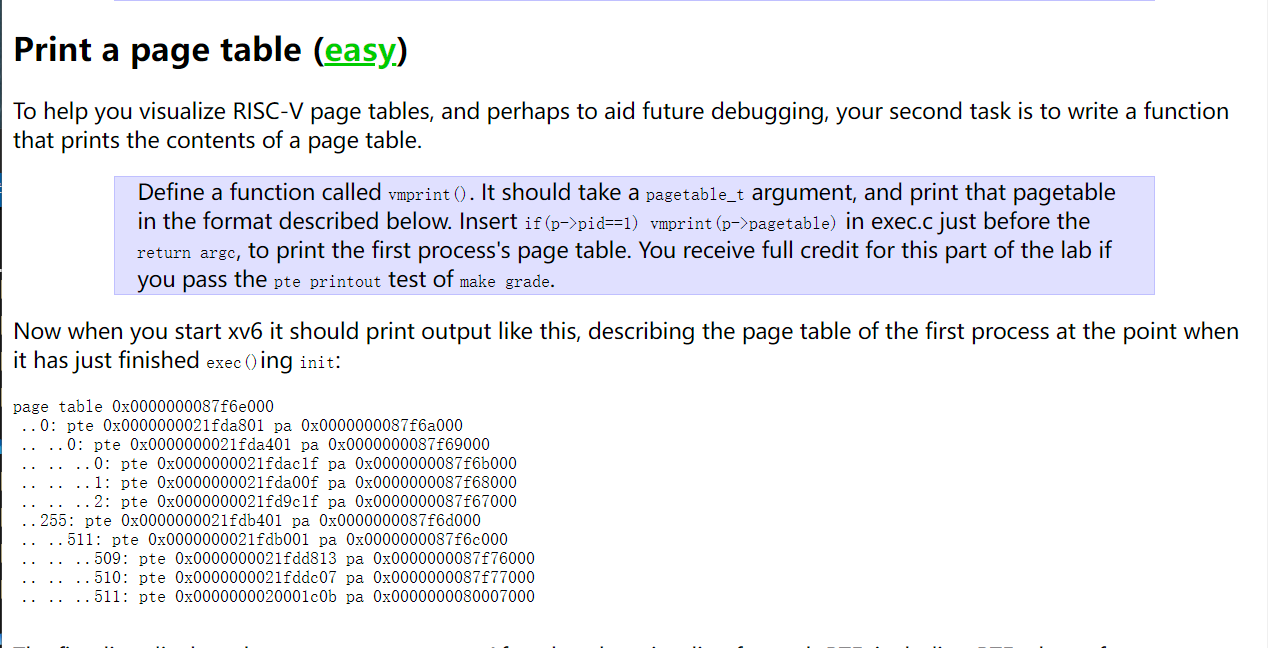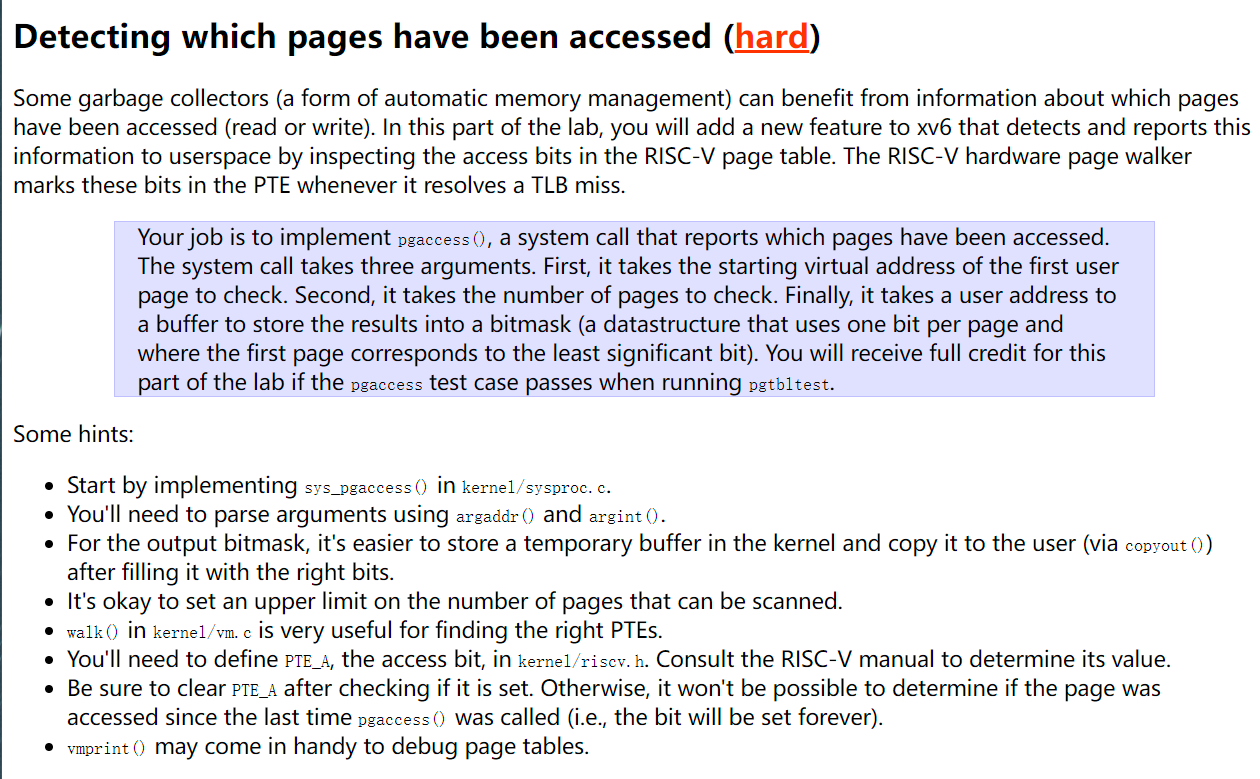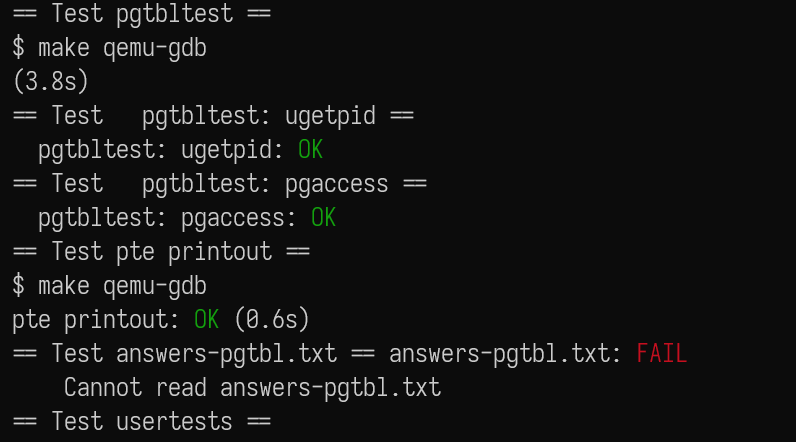upd@2022/9/14：最近把实验的代码放到 github 上了，如果需要参考可以查看这里：

https://github.com/ttzytt/xv6-riscv

# Lab3: page tables

## Speed up system calls// Create PTEs for virtual addresses starting at va that refer to
// physical addresses starting at pa. va and size might not
// be page-aligned. Returns 0 on success, -1 if walk() couldn't
// allocate a needed page-table page.
int
mappages(pagetable_t pagetable, uint64 va, uint64 size, uint64 pa, int perm)
{
// pagetable 是根页表，va 和 pa 分别是虚拟地址起始位置和物理地址起始位置
// perm 是标志位
uint64 a, last;
pte_t *pte;

if(size == 0)
panic("mappages: size");

a = PGROUNDDOWN(va);
last = PGROUNDDOWN(va + size - 1);
// PGROUNDOWN 实际上是把一个数字的后 12 位全部都设成了 0
// 所以 a 表示新映射的起始地址，last 为最后一个要映射的页帧

for(;;){
if((pte = walk(pagetable, a, 1)) == 0)
return -1;
if(*pte & PTE_V)
panic("mappages: remap");
*pte = PA2PTE(pa) | perm | PTE_V;
if(a == last)
break;
a += PGSIZE;
pa += PGSIZE;
// 每次新分配一页
}
return 0;
}

if(mappages(pagetable, TRAMPOLINE, PGSIZE,
(uint64)trampoline, PTE_R | PTE_X) < 0){
uvmfree(pagetable, 0);
return 0;
}

// map the trapframe just below TRAMPOLINE, for trampoline.S.
if(mappages(pagetable, TRAPFRAME, PGSIZE,
(uint64)(p->trapframe), PTE_R | PTE_W) < 0){
// 没映射成功的话会把之前的 unmap，而不是这个本身
uvmunmap(pagetable, TRAMPOLINE, 1, 0);
uvmfree(pagetable, 0);
return 0;
}

uvmfree() 的源码如下：

// Free user memory pages,
// then free page-table pages.
void
uvmfree(pagetable_t pagetable, uint64 sz)
{
if(sz > 0)
uvmunmap(pagetable, 0, PGROUNDUP(sz)/PGSIZE, 1);
freewalk(pagetable);
}

// Recursively free page-table pages.
// All leaf mappings must already have been removed.
void
freewalk(pagetable_t pagetable)
{
// there are 2^9 = 512 PTEs in a page table.
for(int i = 0; i < 512; i++){
pte_t pte = pagetable[i];
if((pte & PTE_V) && (pte & (PTE_R|PTE_W|PTE_X)) == 0){
// this PTE points to a lower-level page table.
uint64 child = PTE2PA(pte);
freewalk((pagetable_t)child);
pagetable[i] = 0;
} else if(pte & PTE_V){ // 重点：PTE_V 为 1，说明映射没取消，会 panic
panic("freewalk: leaf");
}
}
kfree((void*)pagetable);
}

if(mappages(pagetable, USYSCALL, PGSIZE, (uint64)(p->usyscall), PTE_R | PTE_U) < 0){
// 映射完成后，我们访问 USYSCALL 开始的页，就会访问到 p->usyscall
uvmunmap(pagetable, TRAMPOLINE, 1, 0);
uvmunmap(pagetable, TRAPFRAME, 1, 0);
uvmfree(pagetable, 0);
return 0;
}

// Free a process's page table, and free the
// physical memory it refers to.
void
proc_freepagetable(pagetable_t pagetable, uint64 sz)
{
uvmunmap(pagetable, USYSCALL, 1, 0); // 新添加的
uvmunmap(pagetable, TRAMPOLINE, 1, 0);
uvmunmap(pagetable, TRAPFRAME, 1, 0);
uvmfree(pagetable, sz);
}

if((p->trapframe = (struct trapframe *)kalloc()) == 0){
freeproc(p);
release(&p->lock);
return 0;
}

// 分配 usyscall 页
if((p->usyscall = (struct usyscall *)kalloc()) == 0){
freeproc(p->usyscall);
release(&p->lock);
return 0;
}
p->usyscall->pid = p->pid;
// 创建完了顺便把 pid 直接放进去

int
ugetpid(void)
{
struct usyscall *u = (struct usyscall *)USYSCALL;
return u->pid;
}void
vmprint(pagetable_t pagetable, uint dep){
if(dep == 0)
printf("page table %p\n", pagetable);
for(int i = 0; i < 512; i++){
pte_t pte = pagetable[i];
if(pte & PTE_V){
for(int j = 0; j < dep; j++)
printf(".. ");
uint64 child = PTE2PA(pte);
printf("..%d: pte %p pa %p\n", i, pte, child);
if(dep < 2)
// 如果层数等于 2 就不需要继续递归了，因为这是叶子节点
vmprint((pagetable_t) child, dep + 1);
}
}
}

if(p->pid == 1)
vmprint(p->pagetable, 0);

## Detecting which pages have been accessedpagetable_t u_pt = myproc()->pagetable;
uint ck_siz;
try(argint(1, &ck_siz), return -1);
try(argaddr(2, &mask_addr), return -1);Each leaf PTE contains an accessed (A) and dirty (D) bit. The A bit indicates the virtual page has been read, written, or fetched from since the last time the A bit was cleared. The D bit indicates the virtual page has been written since the last time the D bit was cleared.

#define PTE_A (1L << 6) // 左移六位是看上图决定的

if(ck_siz > 32){
return -1;
}

pte_t* fir_pte = walk(u_pt, fir_addr, 0);

for(int i = 0; i < ck_siz; i++){
if((fir_pte[i] & PTE_A) && (fir_pte[i] & PTE_V)){
fir_pte[i] ^= PTE_A; // 复位
}
}

ck_siz 大于 32 的话我们就没有那么多位去在 mask 中储存，所以要返回。

for(int i = 0; i < ck_siz; i++){
if((fir_pte[i] & PTE_A) && (fir_pte[i] & PTE_V)){
fir_pte[i] ^= PTE_A; // 复位
}
}

try(copyout(u_pt, (uint* )mask_addr, &mask, sizeof(uint)), return -1);

## 总结：